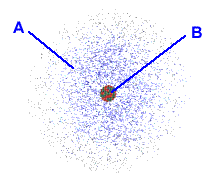# Introductions to Chemistry Chapter 5 Flashcards

Set Details Share
created 10 years ago by bmills5
24,810 views
updated 10 years ago by bmills5
College: First year, College: Second year, College: Third year, College: Fourth year
Subjects:
introduction to chemistry
Page to share:
Embed this setcancel
COPY
code changes based on your size selection
Size:
X

1

True or False:
According to Dalton, atoms of different elements always combine on a one-to-one basis when they form compounds.

False

2

Which of the following applies to the proton?

A) charge = -1

B) charge = +1

C) mass 1 amu

D) charge = 0

E) mass 0 amu

B) charge = +1
C) mass 1 amu

3

True or False:
The mass of a proton is about the same as the mass of an electron.

False

4

True or False:
Most of the alpha particles that were fired into the gold foil in the Rutherford experiment passed straight through the foil.

True

5Choose the region of the atom that accounts for most of its mass

B

6

How many electrons, protons, ans neutrons are there in an atom of tin-117, ^117^Sn?

Protons: 50
Neutrons: 67
Electrons: 50

7

Write the composition of one atom of the ^119^Sn isotope. (electrons, protons, ans neutrons)

Protons: 50
Neutrons: 69
Electrons: 50

8

Write the composition of one atom of the ^28^Si isotope. (electrons, protons, ans neutrons)

Protons: 14
Neutrons: 14
Electrons: 14

9

Fill in the rest;
Element Symbol:
Nuclear Symbol: 35/17 Cl
Atomic Number:
Mass Number:
Protons:
Neutrons:
Electrons in Neutral Atom:

Element Symbol: Cl
Nuclear Symbol: given
Atomic Number: 17
Mass Number: 35
Protons: 17
Neutrons:18
Electrons in Neutral Atom: 18

10

Fill in the rest;
Element Symbol: Cr
Nuclear Symbol:
Atomic Number:
Mass Number: 51
Protons:
Neutrons:
Electrons in Neutral Atom: 24

Element Symbol: Given
Nuclear Symbol: _24_Cr^51^
Atomic Number: 24
Mass Number: Given
Protons: 24
Neutrons: 27
Electrons in Neutral Atom: Given

11

Fill in the rest;
Element Symbol:
Nuclear Symbol:
Atomic Number:
Mass Number:
Protons: 17
Neutrons:20
Electrons in Neutral Atom:

Element Symbol: Cl
Nuclear Symbol: _17_Cl^37^
Atomic Number: 17
Mass Number: 37
Protons: Given
Neutrons: Given
Electrons in Neutral Atom: 17

12

How many protons, neutrons and electrons are there in a neutral atom of the isotope with the nuclear symbol:
_26_Fe^59^

protons:26
neutrons:33
electrons:26

13

How many protons, neutrons and electrons are there in a neutral atom of phosphorus-28?

protons: 15
neutrons: 13
electrons: 15

14

a) What is the mass number of an atom that contains 7 protons, 7 neutrons, and 7 electrons?
b) What is the symbol for the element?

a) mass number = 14
b) element = N

15

a) How many protons and neutrons are there in the nucleus of an atom that has an atomic number of 11 and a mass number of 20?
b) What is the symbol for the element?

a) protons = 11 neutrons = 9
b) element = Na

16

A certain element consists of two stable isotopes.
The first has an atomic mass of 68.9257 amu and a percent natural abundance of 60.40 %.
The second has an atomic mass of 70.9249 amu and a percent natural abundance of 39.60 %.

What is the atomic mass of the element?

69.72 amu

17

The element antimony has two stable isotopes, antimony-121 with an atomic mass of 120.9038 amu and antimony-123 with an atomic mass of 122.9041 amu. From the atomic mass of Sb = 121.75 one can conclude that:

A) antimony-123 has the highest percent natural abundance
B) both isotopes have the same percent natural abundance
C) antimony-121 has the highest percent natural abundance

C. antimony-121 has the highest percent natural abundance

18

A certain element consists of two stable isotopes.
The first has an atomic mass of 120.9038 amu and a percent natural abundance of 57.25 %.
The second has an atomic mass of 122.9041 amu and a percent natural abundance of 42.75 %.
What is the atomic mass of the element?

121.76 amu

19

a) What is the symbol for the element that is in group 5A and period 3?
b) What is the symbol for the element that is in group 4A and period 2?
c) What is the symbol for the element that is in group 1B and period 4?
d) What is the symbol for the element that is in group 1A and period 5?

a) P
b) C
c) Cu
d) Rb

20

Refer to a periodic table. Give the symbol for an element that is:
1) a transtion metal in the fourth period
2) a metal in the third period
3) a non-metal in group 6A, or 7A with Z < 40
4) a main group metal in the 6th period

1) Sc
2) Na
3) O
4) Cs

21

Refer to a periodic table. Which of the following elements are nonmetals?
Choose all that apply.
a) S (Z = 16)
b) Cs (Z = 55)
c) Ar (Z = 18)
d) Fe (Z = 26)
e) None of the Above

A & C